Regression Goodness of Fit

The purpose of modeling is to find the best model that can represent your data. Suppose you have a regression formula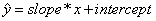as the best line model. How can we be sure that the best line is linear? In other words, how fit is the data to our model? There are unlimited numbers of model combination aside from linear model. Our data may be represented by curvilinear or non-linear model.

The first step is to see visually by plotting the data. Use independent variable as x-axis and dependent variable as y-axis. This plot will give you idea on what type of model you may use as the best-fit model for your data. Modeling is quite an art that we need to 'guess' what is the best model. If the plot shows that the data is not linear, you must try to use other type of model or other combination of variables. Do not force yourself to use linear model when your data is non-linear!

Several indices can be used to examine the goodness of fit of the model. These indices must be used with care and understanding on the meaning. Most common indices are

• R-squared, or coefficient of determination
• Standard Error
• F statistics
• t statistics

To say that your model is fit, you need to prove that all those indices should exceed the criteria. Below is the brief discussion of these indices together with the criteria.

One of the indices to measure model goodness of fit is R-squared, or coefficient of determination. It is the proportion of variation explained by the best line model. It depends on the ratio of sum of square error from the regression model (SSE) and the sum of squares difference around the mean (SST = sum of square total)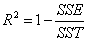where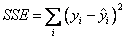and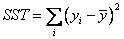.

However, the SST and SSE are not measure of the variance. To use the proportion of variances, we need to average the sum of square. As the result we have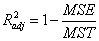Where mean square error is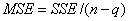and mean square total is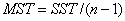for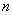is the number of sample and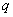is the number of coefficients in the model. Obviously, the relationship of R-squared and adjusted R-squared is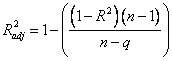. For general rule of thumb, the R-squared or adjusted R-squared should be higher than 0.80 to produce a good linear model. If your R-squared is less than 0.5, it is recommended that you consider other type of model rather than linear model.

Standard Error is another index that often be used for goodness of fit of the model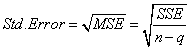Another index for goodness of fit of the model is F-statistic,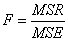where Mean Square Regression is given as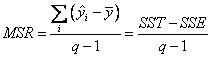The F statistics is often presented as ANOVA (analysis of Variance) table below

 Degree of freedom Sum of square Mean square F Regression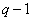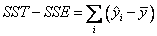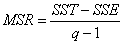Residual (Error)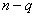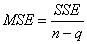Total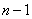If the R-squared approach one, the value of standard error will approach zero and the value of F statistic goes to infinity. The F statistic is compared with the F value from the F distribution with degree of freedom (,). You will see this table in the example.

You may allow some degree of error for your model to be quite small. This error degree is called significant level, denoted by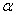. For many practical purposes, we use= 5%. If the significant levelis less than 0.05, the model is said to be best fit. Since the three indices are related to each other, for practical purposes, we often use only R-squared as the index to represent best fit of the model.

While the other four indices above represent the overall fitness of the model, t statistics explain the fitness of individual model parameter. If the t-statistics of a parameter is less than t distribution with degree of freedom n-2 at significant level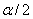, that parameter cannot explain the model well. For practical purposes, when your data is more than n >30 samples, we can use the value of Normal distribution to approximate the t distribution. For significant level= 0.05, you may use threshold of 1.96. Thus, if the t-statistics of a parameter is less than 1.96, that parameter cannot be used to explain the model .

In the next sections , you may see how to obtain our best line model using linear regression formula by hand calculation or spreadsheet. You may apply that formula without worrying about how to compute using the linear regression formula, check how you could do it with just a few clicks and little typing using Microsoft Excel.

Preferable reference for this tutorial is

Teknomo, Kardi (2015) Regression Model using Microsoft Excel. http://people.revoledu.com/kardi/tutorial/Regression/